# Kernel Methods and Support Vector Machines de-Mystified

We give a simple explanation of the interrelated machine learning techniques called kernel methods and support vector machines. We hope to characterize and de-mystify some of the properties of these methods. To do this we work some examples and draw a few analogies. The familiar no matter how wonderful is not perceived as mystical.

## Goals of this writeup

1. De-mystify kernel methods and support vector machines

Kernel methods and support vector machines have taken mythological proportions in the machine learning imagination. Partly this is because a number of good ideas are overly associated with them: support/non-support training datums, weighting training data, discounting data, regularization, margin and the bounding of generalization error. My issue is that these are all important enough ideas to stand on their own and are often seen in simpler settings. The observations that inform my view are as follows:

• Kernel methods and support vector machines are in fact two good ideas. Each is important even without the other: kernels are useful all over and support vector machines would be useful even if we restricted to the trivial identity kernel.
• Small scale “kernel tricks” are not that different than the classic technique of adding “interaction variables.” Kernels let you escape from the limits of “linear hypotheses” (really by moving to a bigger space where things are again linear but look curved from the point of view of your smaller original space). We demonstrate the linear methods and “primal kernel tricks” in A Demonstration of Data Mining.
• Support/non-support is the central issue of nearest neighbor classifiers.
• Weighing training data is most famously shared by logistic regression and boosting.
• Re-weighting of data by smoothing kernels (different but related use of the work “kernel”) is central to non-parametric statistics (kernel smoothers and splines).
• Regularization is of supreme importance in modeling in general.
• Most practitioners get tired of “kernel shopping” and fall back to the identity, cosine or radial/Gaussian kernels.
2. Show concrete examples of what are and what are not kernels.
Few sources give enough theorems to think about kernels abstractly and fewer still work concrete examples.
3. Use as little math as possible (which, unfortunately, turns out to be quite a bit). We will discuss encodings and stopping conditions (important to understand what is going on) but avoid explaining the optimizers (the most beautiful part of support vector machines, but also the part that is available pre-packaged in libraries).
4. Call out common magical thinking and unreasonable expectations associate with kernel methods and support vector machines. This should help the reader be in a better position to “defend their doubts” regarding machine learning promises.
5. Try to place all techniques in a wider context (if it is usable only one place it is a trick, if it is usable multiple places it is a technique).
6. Discuss margin and its impact on generalization error.

Generalization error is an effect of “over fitting” where a model has learned things that are true about the training examples that do not hold for the overall truth or concept we are trying to learn (i.e. don’t generalize). Generalization error is the excess error rate we observe when scoring new examples versus the error-rate we saw in learning the training data. Margin is in fact a posterior observation. That is: margin is observed after the training data is seen, not known before data is seen (like, for example, VC dimension). Margin is useful as it bounds generalization error but it is not the prior bound it is often portrayed as. So we assert margin estimates are not much more special than simple cross-validation estimates which can also be performed once we have data available.

The goals are not to try to indict or try to cut down kernel methods or support vector machines, but just to dump some of the associated baggage so they can be used fluidly and without anxiety.

## Example Problem

Consider the following simple (caricature) machine learning problem: we are given a number of points labeled as circles and square and we want to, given a new point, predict the label. In our example the only input will be two numbers: x and y. This example is simple enough that we can depict the entire situation in Figure 1 below: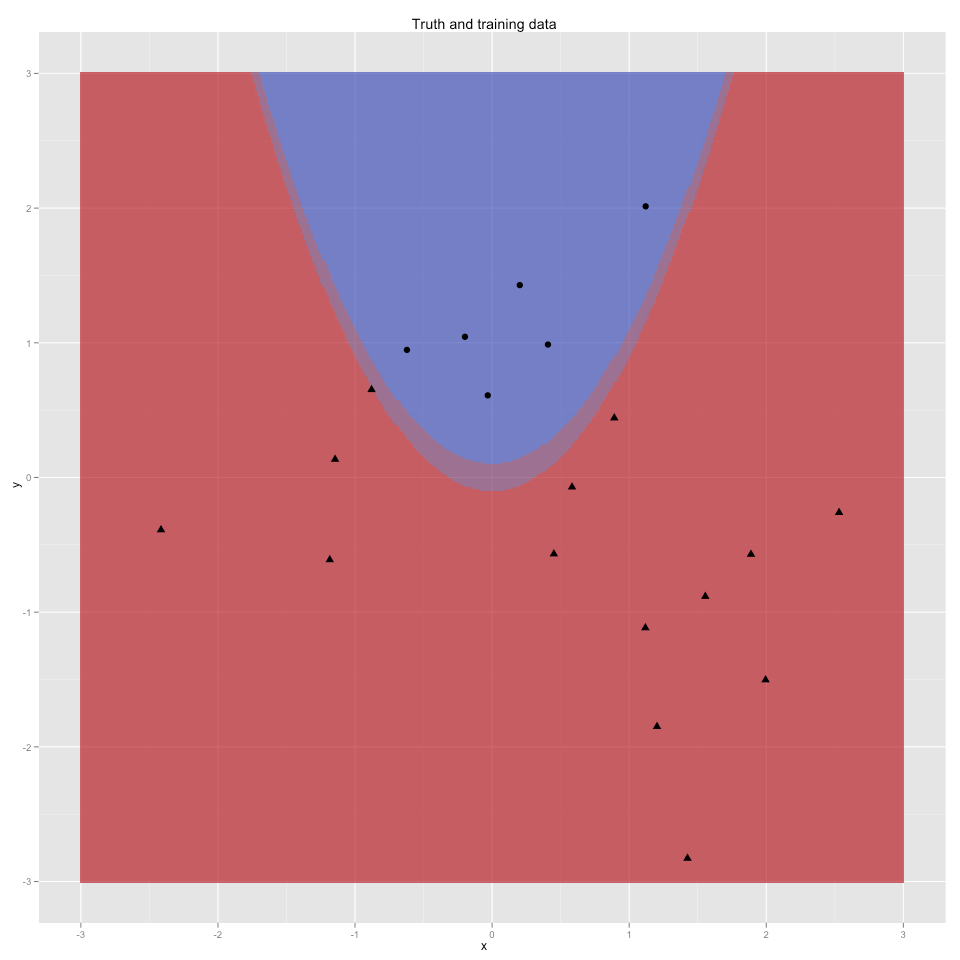Figure 1: Truth and Training Data

We are assuming (for simplicity of exposition) that we have the incredible luck that the label is indeed a deterministic function of x and y. We portray this “ground truth” as the blue parabola- every point found in this region will be considered to be labeled as “circle” and every other point (i.e. the red region) will be labeled as “triangle.” We have overlaid 20 example points (with appropriate shape labels). These points will be our training data. We will try to learn from the observed training data a good approximation of the unseen blue and red regions. This process is called learning or training and the ability to correctly predict the label of new points is called generalization. To make sure our concept is learnable we are going to further guarantee a moat (or margin) the distribution we are picking training and test examples from will never pick a point from the shaded region between the blue and black. That is we will never be asked about an example where x*x is very near y.

To promote visual thinking we are going to avoid formulas until the section titled “precise functions used” (where we will list the formulas used for each graph).

## Nearest Neighbor Solution

An interesting model in these days of big data and fast machines is the nearest neighbor model. Such a model colors each point in space blue or red as it thinks the point should be classified. In this case each point is colored the color given by the closest known training point. This induces the type of model seen in Figure 2 whose boundary is piecewise line segments mid-way between training points. The data points that determine segments of the classification boundary in this way are thought to support the boundary and are called “support examples” or “support vectors.” In fact any training point that is not “supporting” part of the boundary is “irrelevant” (could be removed and we would get the exact same model). This split between important and non-important training points is considered one of the important observations from the theory of support vector machines, but we see it even here in the nearest neighbor models.Figure 2: 1 nearest neighbor model

Notice that the nearest neighbor model in Figure 2 is “not half bad.” The blue region is much too wide, but near the training data the blue mass is roughly in the correct position. If we had infinite data and infinite computational resources this sort of model would be hard to beat (as any point we are likely to be tested on would have a lot of very near examples to work from). We can try to clean up the shape of the model a bit by using a vote of the 2 nearest points to color each point in the plane (see Figure 3) or even the majority of the 3 nearest points (see Figure 4).Figure 3: 2 nearest neighbor model

The purple region in Figure 3 is a “region of uncertainty” where net-vote over the 2 nearest points is zero (they gave inconsistent advice). We can use this as a hint that predictions take from this region are less reliable. As you may notice, we are not getting real improvements. We should always spend more time getting more features (useful coordinates in addition to x and y) and more data; and spend less time tweaking models. But if your data and features are fixed all you work on is your modeling technique (alternately: your modeling technique is all you can prepare before receiving features and data). So we will continue to discuss features and technique.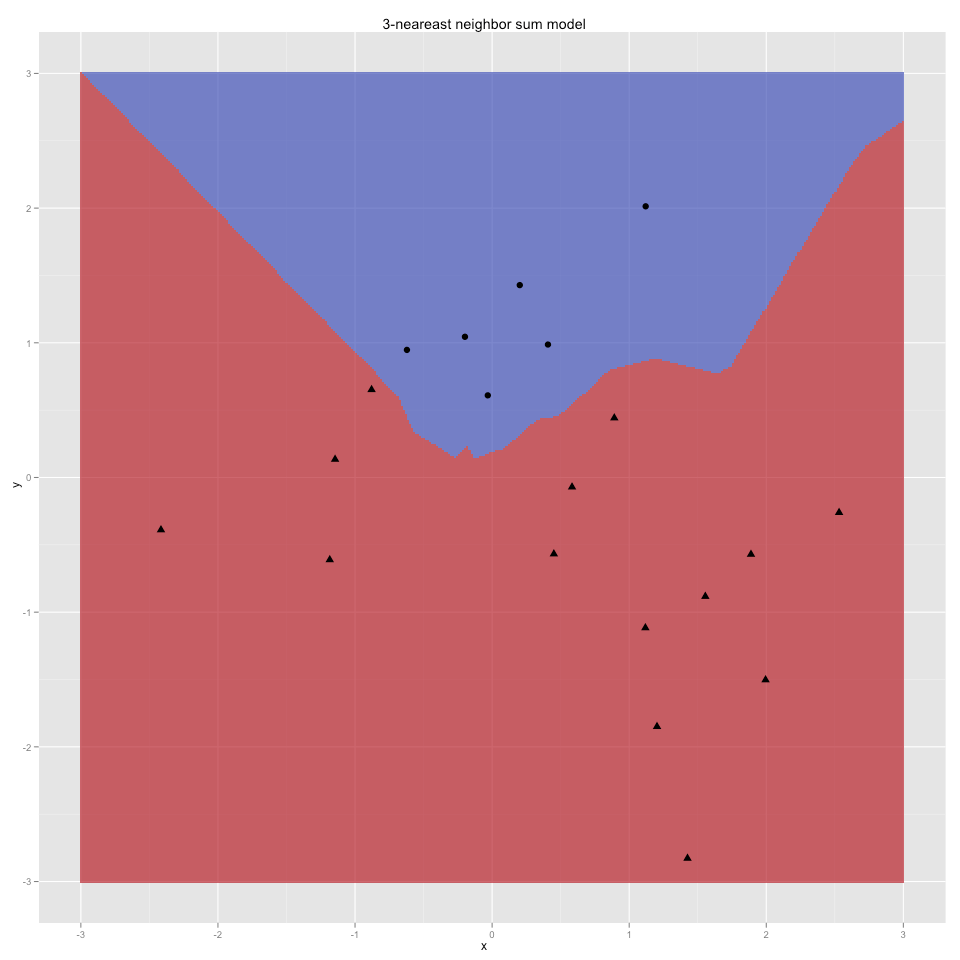Figure 4: 3 nearest neighbor model

Nearest neighbor classifiers are optimal in the sense that with an infinite amount of data the 1-nearest neighbor classifier has an error rate that approaches twice the Bayes error rate (the Bayes error rate being the ideal error observed on identical repetitions, or the theoretical best error rate) and for large k the k-nearest neighbor method approaches the Bayes error rate itself (see for example k-nearest neighbor algorithm ).

However the effectiveness of the nearest neighbor classifiers is coming from the very low dimension (2) of our input variables x and y. If we were attempting to predict from n variables we might need an amount of data exponential in n to get a useful nearest neighbor classifier. This is an inefficient use of data of the data; what we want is to use all of the data we have effectively. To do this we further assume there is some relational reason that the position of the point in the plane determines the shape-label (i.e. that we are learning, generalizing, interpolating and extrapolating- not just memorizing). We set our ambitions above memorizing and move towards functional modeling. We start thinking in terms of change: how the label density of examples changes as we move is a good clue as to how it will continue to change. We can do this either in a parametric or primal formulation (where we attempt to directly infer parameters of an assumed functional form as in regression or logistic regression) or in a non-parametric or dual form (where we attempt to learn relations between points and the training data instead of parameters). We have discussed this before ( A Demonstration of Data Mining ) and expanded on primal methods ( Learn Logistic Regression (and beyond, The Simpler Derivation of Logistic Regression and The equivalence of logistic regression and maximum entropy models).

## Functional Solution

Our next idea is: can we use our training data to tease out a functional form for the relation between x,y and shape label? The first function we will try is chosen to be similar to the nearest neighbor model we just demonstrated. For this form we say each training point is the center of a Gaussian hump (say up for blue/circle and down for red/triangle) which we will call a “discount function” (or informally a hump). A “Gaussian” is just a function that falls off exponentially in the square of Euclidian distance from a central point (we can see the simple form of such functions in the “precise functions used” section). The contour lines (or levels as seen from looking down upon) of one such hump or discount function are shown in figure 5 (all graphs from here on in will be looking down at a height represented by color and contour lines, much like topographic maps).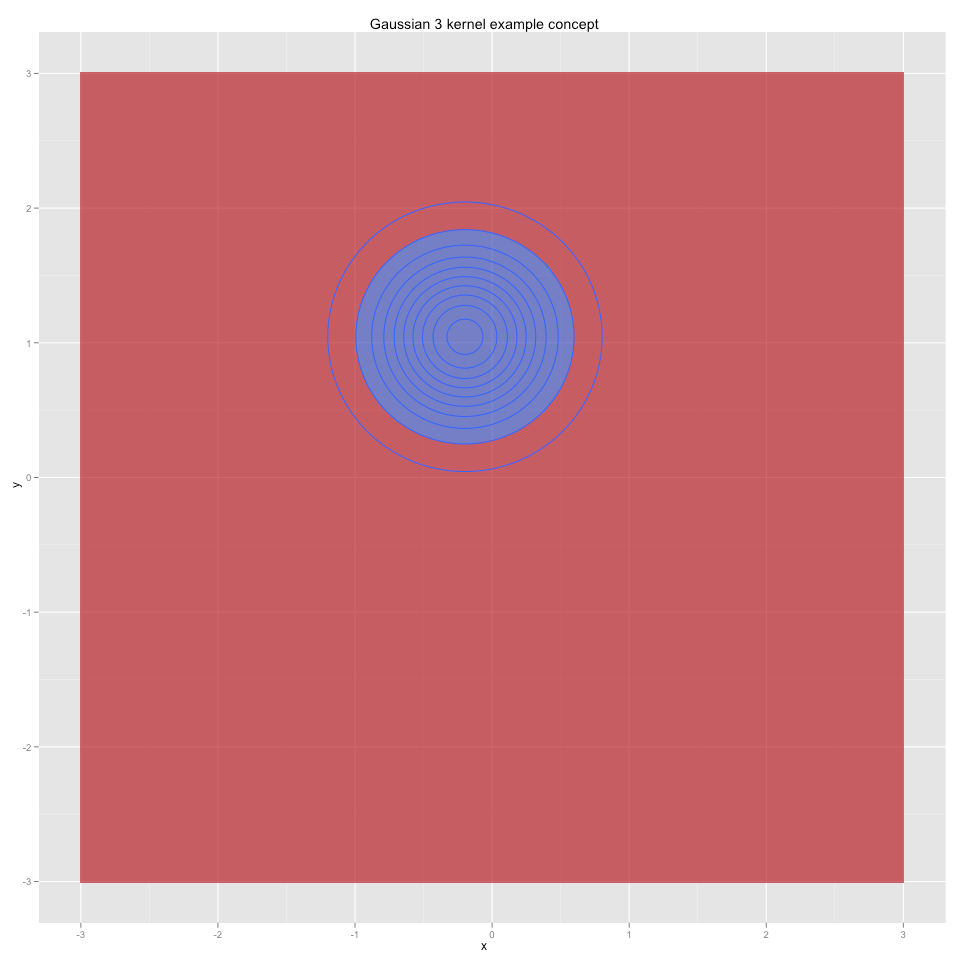Figure 5: Narrow Gaussian example concept

This particular discount function has its maximum (or peak) centered on a data point and then rapidly falls as we move away from the training point. The only property we will use in this section is that we can evaluate the discount easily (so we don’t at this time need or make use of any restrictive properties like integrability, positive semi-definiteness or even anything like bounded level sets).
We could make our functional model just the sum of all of these up and down humps over all of the training data. Each point gets a hump of the same height and same radius, pointing up for blue/circle and down for red/triangle. The net coloring of this sum function is shown in figure 6.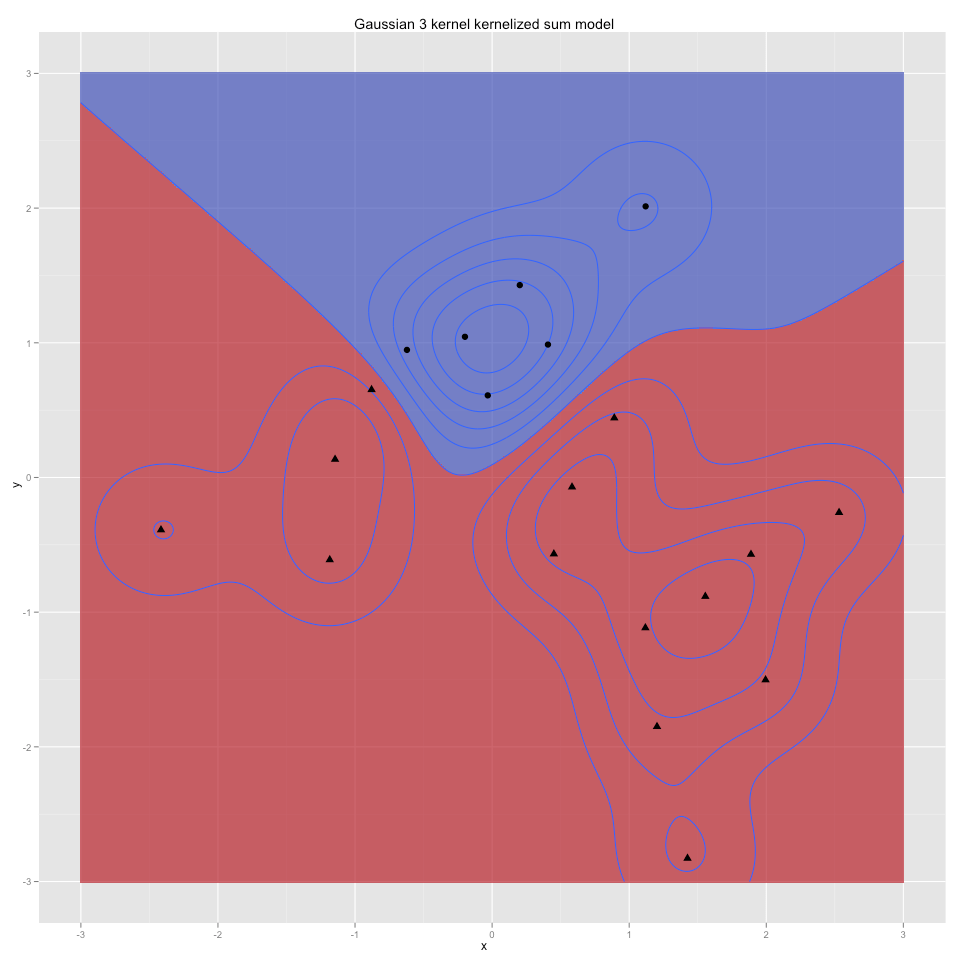Figure 6: Narrow Gaussian sum model

The idea is that this would imitate the nearest neighbor model we have already seen. This is because even though we are summing over all data the closest training examples should usually dominate (since the Gaussian humps we picked fall to zero as we get further from their centers). The correspondence to nearest neighbor increases if we tighten the radius of our functions (searching for the right radius is a very important statistical problem called “bandwidth estimation”). Figure 7, for example shows the sum over much narrower Gaussians.Figure 7: Very narrow Gaussian sum model

Figure 7 looks even more like the nearest neighbor solution. In fact your could think of the discount-weighted sum over all of the data as the natural model (as it is easer to reason about) and the nearest neighbors a more efficient approximation (summing only over near points). We also have seen that we can alter the smoothness of our model (which has consequences on model generalization) by changing the steepness of our discount functions. We can also build intermediate models (such as building a nearest neighbor but using discount weighted sums instead of uniform voting in the neighborhoods). We haven’t yet greatly improved on nearest neighbor, but we have identified a couple of obvious avenues for further improvement: mess with the bandwidths (the obvious idea to try and deal with near/far scale) or re-weight the data (as one of the lessons of the nearest neighbor model is that points that are not near a boundary are less important).

## Bandwidth Solution

As a digression lets play with bandwidth a bit first. Suppose simultaneously for each training datum we picked an ideal bandwidth or steepness of the Gaussian.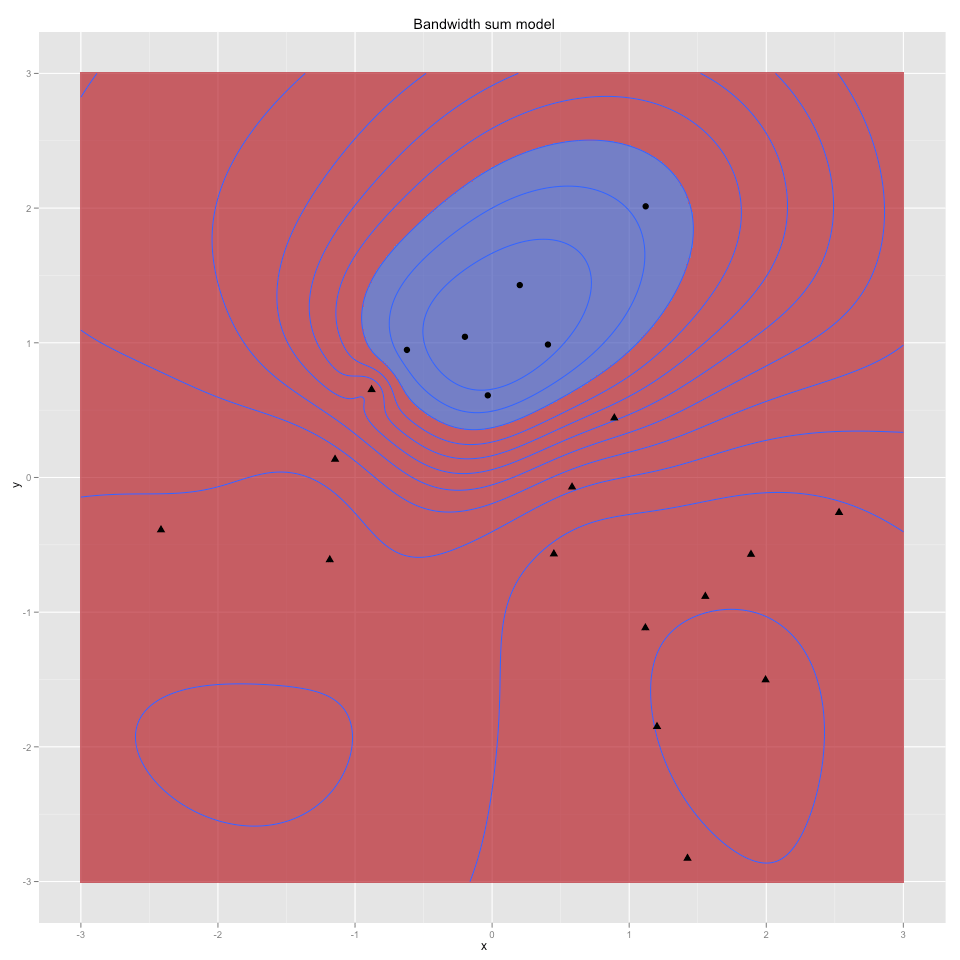Figure 8: Simultaneous bandwidth model

This simultaneous bandwidth model depicted in figure 8 was determined by picking a simultaneous assignment of Gaussian widths (each training datum gets its own width) that maximizes the log-sum of the category signed model function on the training data (much like the logistic regression maximum likelihood decoding). This differs from typical bandwidth selection problems where all points are given a single common “best bandwidth.” We instead picked a different bandwidth for each point (bandwidths picked to maximize how much of the correct category portion of the sum was correct on each training example). The blue region is reasonable, but does not match the shape of the true concept. Partly this is because there has not been enough training data to have learned a lot about the true shape (for example there is no empirical evidence for circle/blue in the top-left quadrant). The other reason is a bias inherent to this type of model. If one of the bandwidth functions is wider than all of the others (which is almost certain to occur) then it falls slower than all of the others and for points very far away from the center of the training data this one function is most of what remains. Or equivalently: due to the nature of this modeling technique one of the concepts learned is going to be the union of bounded islands and the other concept will grab all points sufficiently far away from the center of the training data. This is strong (and undesirable) bias, but it is a bias also shared by support vector machines with Gaussian kernels (though support vector machines get it from their so-called “dc-term” b not being zero; this will be discussed later). Our bandwidth correction was successful, but frankly the optimization problem we solved to estimate the optimal bandwidths was nasty and would not be something we would advise for a large amount of data.

## Data weighting solution (support vector machine)

So instead of messing with the bandwidths let us consider re-weighting the Gaussians in our sum. We will leave the shape of each Gaussian the same but allow each individual training datum to have a unique weight or importance. Perhaps by picking the right weights we can get a better model. By “best” we will mean “best margin” (to be defined later) because with “best margin” as our objective the optimization problem of solving for the best data weights has a particularly beautiful form that can be reliably solved at great scale. This is called a “support vector model” (or support vector machine) and we will describe these ideas in detail later. But first lets see the result. Figure 9 shows the original narrow Gaussians re-summed according to the weights picked by the support vector method (instead of all being 1 as in figure 6).Figure 9: Narrow Gaussian support vector model

Figure 9 is again an improvement. The figure is formed by taking a signed sum of our discount functions centered at our training points and weighted by the “support vector machine weights.” The signs are picked with one class encoded as +1 and the other as -1. The colors red and blue are picked if the sum is above or below a constant “b” called “the dc-term” (part of the support vector solution). The solution again looks okay: all the training points are in the correct color region (and you can’t hope to interpolate let alone extrapolate if you can’t even reproduce your training concept). Also, as with the bandwidth model, contours are set sensibly (steep/dense where categories are near each other, shallow/sparse where things are safe). We see the same inductive bias: one of the concepts is the union of bounded islands (this will always going to be the case when the model picks b != 0). This bias is not obvious from the kernel choice, as it is hidden in the dc-term of the support vector fitting equations (it is not part of the kernel, but can be defeated by choosing unbounded kernels). An interesting empirical fact about support vector models is they tend to work well with a larger bandwidth. For example if we use a wider Gaussian we get an even more convincing model (see Figure 10).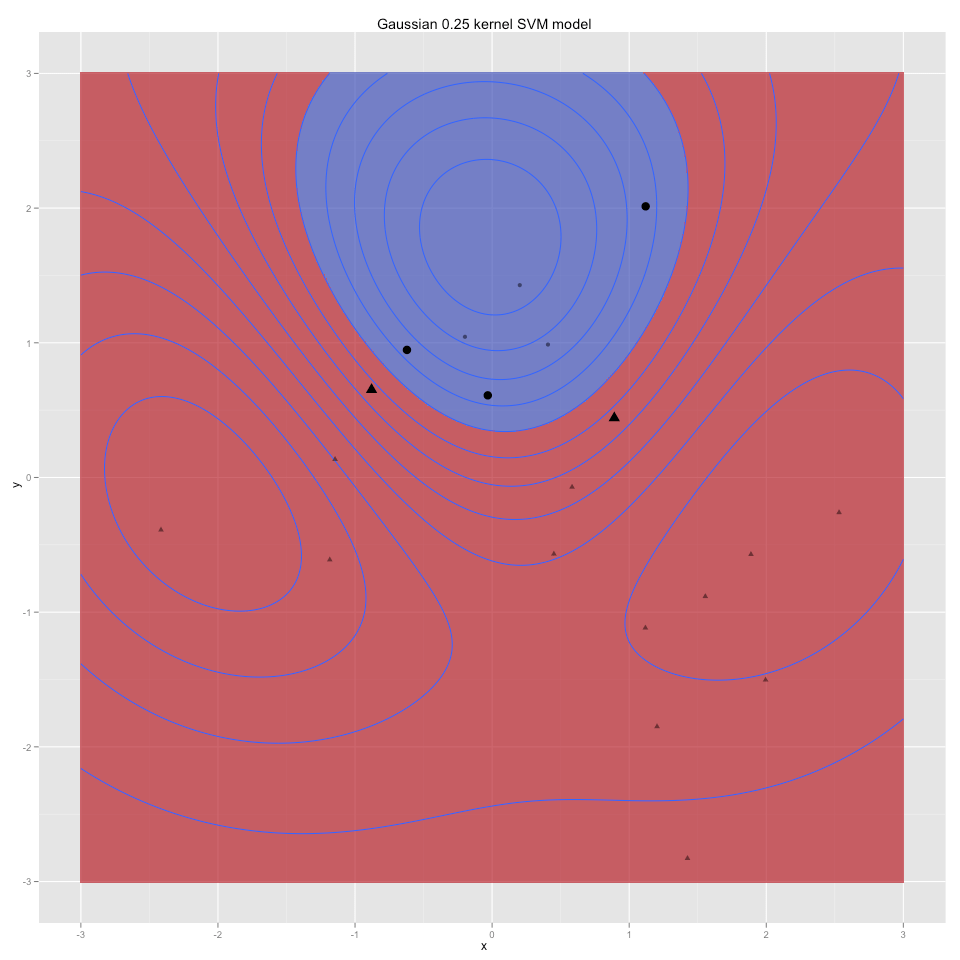Figure 10: Wide Gaussian support vector model

In figure 10 we have also called out one of the important features of support vector machines. In the literature all datums are called “vectors” (as they are represented in coordinates) and a subset of these are called the support vectors. In figure 10 we have drawn the 4 training examples that turn out to be support vectors as large shapes and all other training examples as small shapes. The support vectors are the datums with non negligible weights. The learned model is a function of the support vectors only. So after fitting we can discard the other 16 training points. This is similar to the fact that nearest neighbor also only needs the training examples that are supporting its model boundary.

Notice that at this point the support vector models are not “magically” better, they in fact look less like the truth we are trying to learn than either the nearest neighbor models or the un-weighted sum models. To fix this we need to do some “kernel shopping” or find functions that better respect what we are trying to model. With the right functions support vector machines can do a very good job at learning the concept (but knowing “the right functions” is a huge hint as to the what the concept is. There is nothing wrong with using a hint but we do have to a method to produce the hint or have a reasonable number of hints to try from.

The “sum over everything with the same weight” solutions from the earlier section are very similar to Naive Bayes which also sums over all matching features with no weight adjustment. The support vector “use the same model but pick better weights” stands over these sum models in very much the same way Logistic Regression stands over Naive Bayes. In fact the optimization problems are very related. If we consider weights over features or coordinates as “primal” and weights over data items as “dual” we can informally say something like: support vector machines optimize by working over dual variables and inspecting for primal weights, and logistic regression works over primal variables and inspects for data weights. But this is a bit vague.

At this point we need to get a bit more explicit and precise.

## Precise functions used

In general we will write our training data as a sequence of n-vectors. Our points will be named u(1) … u(m). For each i = 1..m we also know which category the training point is labeled with. We will encode this as y(1) … y(m) where each y() is either +1 or -1 depending on the training label. So the example we have been working has n=2 and m=20. Let z represent a n-vector we wish to classify.

Figure 6 was a picture of the sign of the function: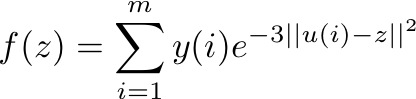Figure 7 was a picture of the sign of the function:Figure 8 was a picture of the sign of the function: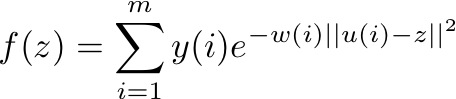where the w(i) are non-negative numbers picked so that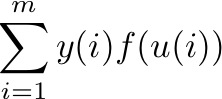is large.

Figure 9 was a picture of the sign of -b plus the function: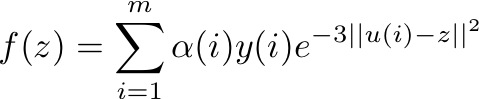where the a(i) and b are picked so “the margin” (discussed later) is large.

Figure 10 was a picture of the sign of -b plus of the function:where the a(i) and b are picked so the margin is large.

All of this repetition is to emphasize the commonality of the models. They are all of the form: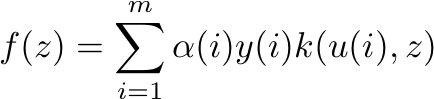where the a(i) are non-negative numbers called the “support weights” and k(,) is a function mapping pairs of vectors to numbers and is called a “a kernel.” Unfortunately there are many incompatible uses of the word kernel in mathematics and statistics. Here “kernel” is being used in the sense of positive semi-definiteness or that k(u,u) ≥ 0 for all u. Note that the support vector machine instead of using the sign of f(z) as its decision instead uses which side f(z) is of a constant b as its category decision. A consequence is: for support vector machines if b is non-zero (as it almost surely will be) and the kernels all go to zero as we approach infinity fast enough (as they are designed to do) then exactly one of the learned classes is infinite and the other is a union of islands (regardless if this was true for the training data).

A lot of awe and mysticism is associated with kernels, but we think they are not that big a deal. Kernels are a combination of two good ideas, they have one important property and are subject to one major limitation. It is also unfortunate that support vector machines and kernels are tied so tightly together. Kernels are the idea of summing functions that imitate similarity (induce a positive-definite encoding of nearness) and support vector machines are the idea of solving a clever dual problem to maximize a quantity called margin. Each is a useful tool even without the other.

The two good ideas are related and unfortunately treated as if they are the same. The two good ideas we promised are:

1. The “kernel trick.” Adding new features/variables that are functions of your other input variables can change linearly inseparable problems into linearly separable problems. For example if our points were encoded not as u(i) = (x(i),y(i)) but as u(i) = (x(i),y(i),x(i)*x(i),y(i)*y(i),x(i)*y(i))
2. we could easily find the exact concept ( y(i) > x(i)*x(i) which is now a linear concept encoded as the vector (0,1,-1,0,0).

3. Often you don’t need the coordinates of u(i). You are only interested in functions of distances ||u(i)-u(j)|^2 and in many cases you can get at these by inner products and relations like ||u(i)-u(j)||^2 = <u(i),u(i)> + <u(j),u(j)> – 2<u(i),u(j)> .

We will expand on these issues later.

The important property is that kernels look like inner products in a transformed space. The definition of a kernel is: there exists a magic function phi() such that for all u,v: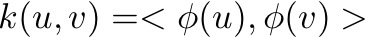.

This means that k(.,.) is behaving like an inner product in some (possibly unknown) space. The important consequence is the positive semi-definiteness, which implies k(u,u)≥0 for all u (and this just follows from the fact about inner products over the real numbers that <z,z>≥0 for all z). This is why optimization problems that use the kernel as their encoding are well formed (such as the optimization problem of maximizing margin which is how support vector machines work). You can “regularize” optimization problems with a kernel penalty because it behaves a lot like a norm. Without the positive semidefinite property all of these optimization problems would be able to “run to negative infinity” or use negative terms (which are not possible from a kernel) to hide high error rates. The limits of the kernel functions (not being able to turn distance penalties into bonuses) help ensure that the result of optimization is actually useful (and not just a flaw in our problem encoding).

And this brings us to the major limitations of kernels. The phi() transform can be arbitrarily magic except when transforming one vector it doesn’t know what the other vector is and phi() doesn’t even know which side of the inner product it is encoding. That is kernels are not as powerful as any of the following forms:

• snooping (knowing the other):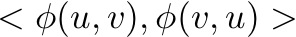• positional (knowing which part of inner product mapping to):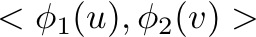• fully general: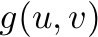Not everything is a kernel.

Some non-kernels are:

1. k(u,v) = -c for any c>0
2. A consequence of the fact that no negative number can be written as a sum of squares or as the limit of a sum of squares in the reals.

3. k(u,v) = ||u-v||

This can be shown as follows. Suppose k(u,v) is a kernel with k(u,u) = 0 for all u (which is necessary to try and match ||u-v|| as ||u-u|| = 0 for all u). By the definition
of kernels k(u,u) = < phi(u), phi(u) > for some real vector valued function phi(.). But, by the properties of the inner real inner product <.,.>, this means phi(u) is
the zero vector for all u. So k(u,v) = 0 for all u,v and does not match ||u-v|| for any u,v such that u ≠ v.

There are some obvious kernels:

1. k(u,v) = c for any c ≥ 0 (non-negative constant kernels)
2. k(u,v) = < u , v > (the identity kernel)
3. k(u,v) = f(u) f(v) for any real valued function f(.)
4. k(u,v) = < f(u) , f(v) > for any real vector valued function f(.) (again, the definition of a kernel)
5. k(u,v) = transpose(u) B v where B is any symmetric positive semi-definite matrix

And there are several subtle ways to build new kernels from old. If q(.,.) and r(.,.) are kernels then so are:

1. k(u,v) = q(u,v) + r(u,v)
2. k(u,v) = c q(u,v) for any c ≥ 0
3. k(u,v) = q(u,v) r(u,v)
4. k(u,v) = q(f(u),f(v)) for any real vector valued function f(.)
5. k(u,v) = lim_{k-> infinity} q_k(u,v) where q_k(u,v) is sequence of kernels and the limit exists.
6. k(u,v) = p(q(u,v)) where p(.) is any polynomial with all non-negative terms.
7. k(u,v) = f(q(u,v)) where f(.) is any function with an absolutely convergent Taylor series with all non-negative terms.

Most of these facts are taken from the excellent book: John Shawe-Taylor and Nello Cristianini’s “Kernel Methods for Pattern Analysis”, Cambridge 2004. We are allowing
kernels of the form k(u,v) = < phi(u), phi(v) > where phi(.) is mapping into an infinite dimensional vector space (like a series). Most of these facts can be checked by
imagining how to alter the phi(.) function. For example to add two kernels just build a larger vector with enough slots for all of the coordinates for the vectors encoding
the phi(.)’s of the two kernels you are trying to add. To scale a kernel by c multiply all coordinates of phi() by sqrt(c).

Multiplying two kernels is the trickiest. Without loss of generality assume q(u,v) = < f(u) ,f(v) > and r(u,v) = < g(u), g(v) > and f(.) and g(.) are both mapping into the same finite dimensional vector space R^m (any other situation can be simulated or approximated by padding with zeros and/or taking limits). Imagine a new vector function p(.) that maps into R^{m*m} such that p(z)_{m*(i-1) + j} = f(z)_i g(z)_j . It is easy to check that k(u,v) = < p(u) , p(v) > is a kernel and k(u,v) = q(u,v) r(u,v). (The reference proof uses tensor notation and the Schur product, but these are big hammers mathematicians use when they don’t want to mess around with re-encoding indices).

Note that one of the attractions of kernel methods is that you never have to actually implement any of the above constructions. What you do is think in terms of sub-routines (easy for computer scientists, unpleasant for mathematicians). For instance: if you had access to two functions q(.,.) and r(.,.) that claim to be kernels and you wanted the product kernel you would just, when asked to evaluate the kernel, just get the results for the two sub-kernels and multiply (so you never need to see the space phi(.) is implicitly working in).

This implicitness can be important (though far too much is made of it). For example the Gaussians we have been using throughout are kernels, but kernels of infinite dimension, so we can not directly represent the space they live in. To see the Gaussian is a kernel notice the following:.

And for all c ≥ 0 each of the three terms on the right is a kernel (the first because the Taylor series of exp() is absolutely convergent and non-negative and the second two are the f(u) f(v) form we listed as obvious kernels). The trick is magic, but the idea is to use the fact that Euclidian squared distance breaks nicely into dot-products ( ||u-v||^2 = < u, u &gt + < v, v &gt – 2 < u , v >) and exp() converts addition to multiplication. It is rather remarkable that kernels (which are a generalization of inner products that induce half open spaces) can encode bounded concepts (more on this later when we discuss the VC dimension of the Gaussian).

## Back to Support Vector Machines

We can now restate what a support vector machine is: it is a method for picking data weights so that the modeling function for a given kernel has a maximum margin. The margin is the minimal distance between a training example and the model’s boundary between blue and red. Notice the 4 support vectors in figure 10 are all not “tight up against the boundary,” but are all the same (minimal distance) from the boundary. This distance is called the margin and the area near the boundary is obviously a place the model has uncertainty (so it makes sense to keep the boundary as far as possible from the training data). The great benefit of the support vector machine is that with access only to the data labels and the kernel function (in fact only the kernel function evaluated at pairs of training datums) the support vector machine can quickly solve for the optimal margin and data weights achieving this margin.

For fun lets plug in new kernel called the cosine kernel:(c ≥ 0).

This kernel can be thought of as having a phi(.) function that takes a vector z and adds an extra coordinate of sqrt(c) and then projects the resulting vector onto the unit sphere. This kernel induces concepts that look like parabolas, as seen in figure 11.Figure 11: Cosine example concept

Figure 12 shows the sum-concept (add all discount functions with same weight) model. In this case it is far too broad (averaging of the cosine concepts which are not bounded concepts like the Gaussians) creates an overly wide model. The model not only generalizes poorly (fails to match the shape of the truth diagram) it also gets some of its own training data wrong.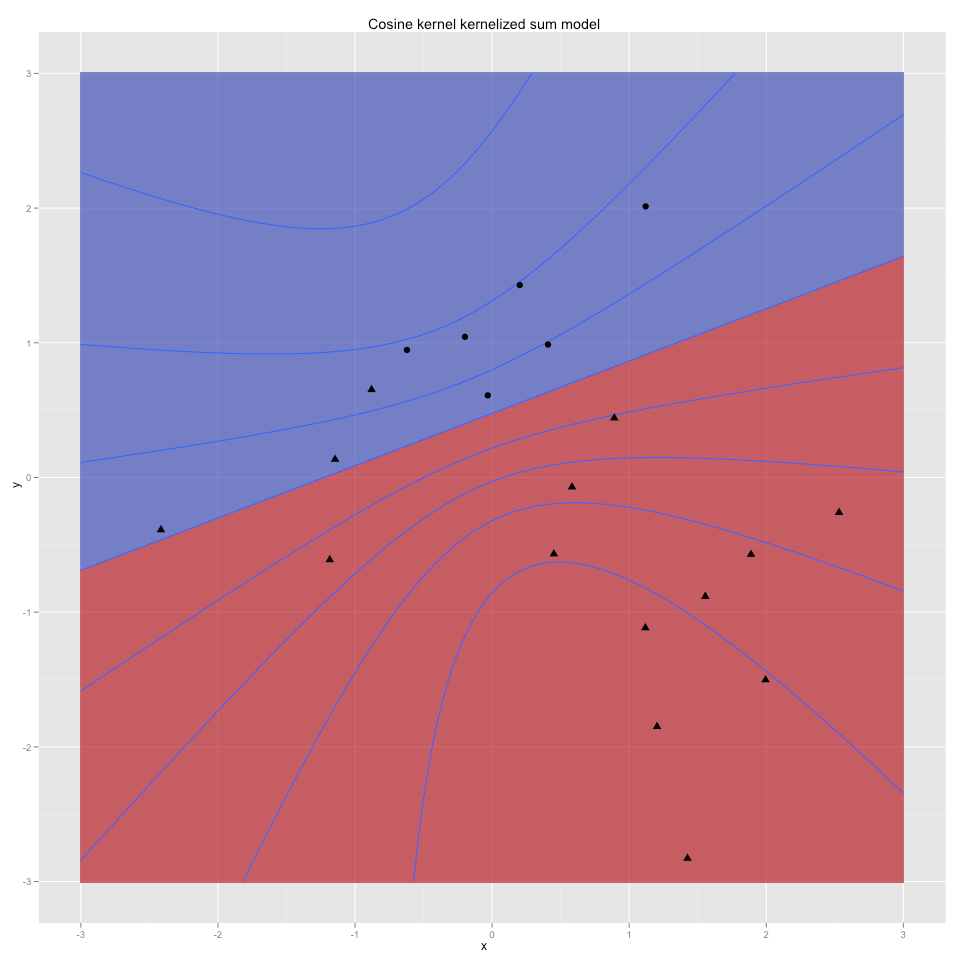Figure 12: Cosine kernel sum model

And figure 13 shows the excellent fit returned by the support vector machine. Actually an excellent fit determined by 4 support vectors (indicated by larger labels). Also notice the support vector machine using unbounded concepts generated a very good unbounded model (unbounded in the sense that both the blue and red regions are infinite). By changing the kernel or discount functions/concepts we changed the inductive bias. So any knowledge of what sort of model we want (one class bounded or not) should greatly influence our choice of kernel functions (since the support vector machine can only pick weights for the kernel functions, not tune their shapes or bandwidths).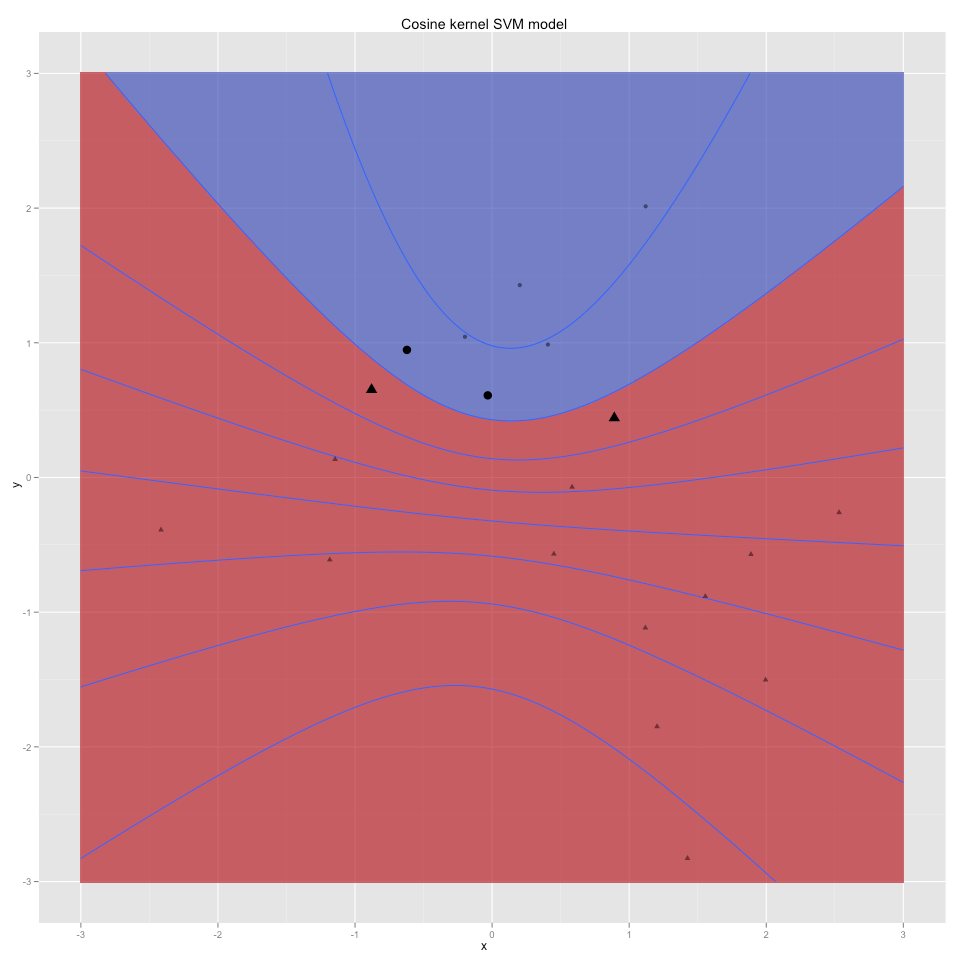Figure 13: Cosine Support Vector model

Another family of kernels (which I consider afflictions) are the “something for nothing” kernels. These are kernels of the form:or higher powers or other finishing functions. The idea is that if you were to look at the expansion of these kernels you would see lots of higher order terms (powers of u_i and v_j)
and if these terms were available to the support vector machine it could use them. It is further claimed that in addition to getting new features for free you don’t use up degrees
of freedom exploiting them (so you cross-validate as well as for simpler models). Both these claims are fallacious- you can’t fully use the higher order terms because they are entangled with other terms that are not orthogonal the outcome and the complexity of a kernel is not quite as simple as degrees of freedom (proofs about support vector machines are stated in terms of margin, not in terms of degrees of freedom or even in terms of VC dimension). The optimizer in the SVM does try hard to make a good hypothesis using the higher order terms- but due to the forced relations among the terms you get best fits like figure 14.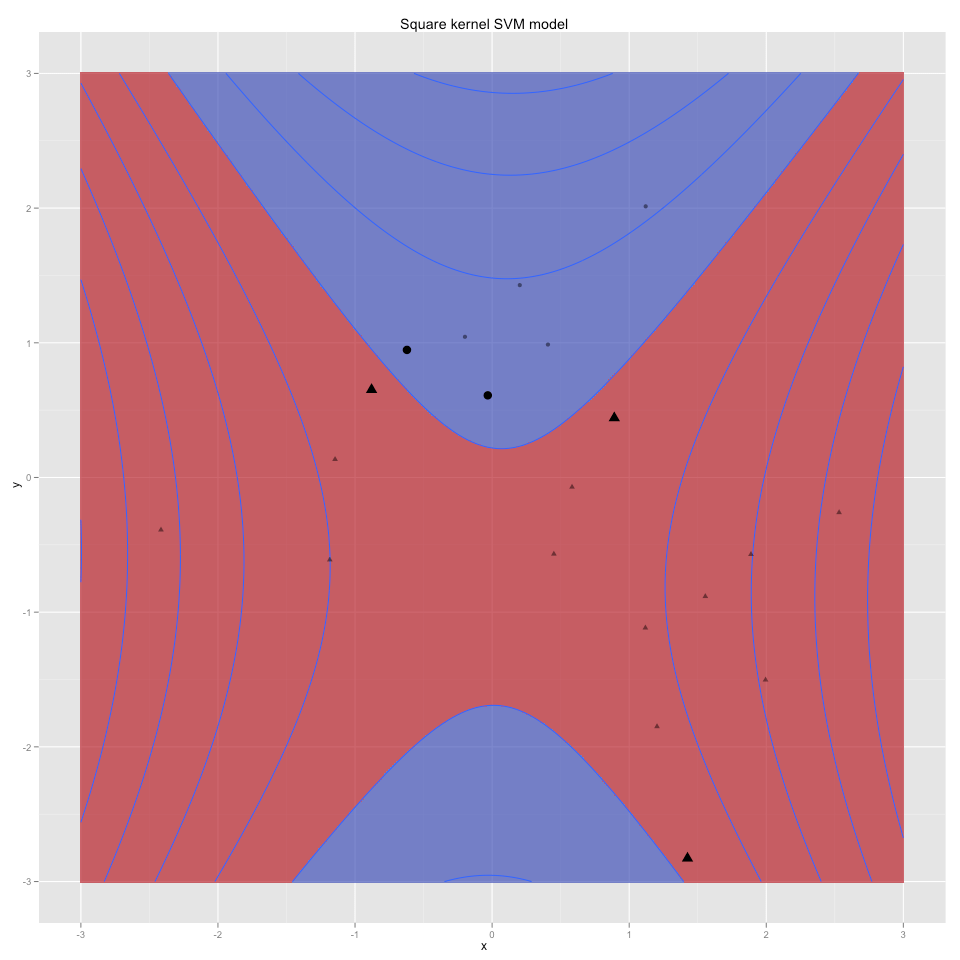Figure 14: Squared magic kernel support vector model

If you want higher order terms I feel you are much better off performing a primal transform so the terms are available in their most general form. That is re-encode the vector u = (x,y) as the larger vector (x,y,x*y,x*x,y*y). You have to be honest: if you are trying to fit more complicated functions you are searching a larger hypothesis space so you need more data to falsify the bad hypotheses. You can be efficient (don’t add terms you don’t think you can use as they make generalization harder) but you can’t get something for nothing (even with kernel methods).

## Back to margin

Large margin (having a large distance between the decision boundary and all training examples) is a good idea. At the simplest level it means anything close to a training data point gets classified correctly (so the model is immune to an amount of fuzz proportional to the margin width).

We get the following remarkable generalization theorem (this one is the hard SVM version, we weaken the result a bit to make it easier to state). Suppose we train a model using kernel k(.,.) on m training examples u(1),…,u(m) with training labels y(1)…y(1). Further assume the hidden true concept is separable with respect to our kernel and data distribution with a margin of at least w (that is for any sample we draw we can find a SVM model that classifies all of the training data correctly and sees a margin of at least w). Or more simply: assume w is behaving like a constant with respect to m (i.e. it is not going down as we increase sample size). Then with probability at least 1-d the generalization error (that is error seen on new test points not seen during training, but drawn from the same distribution as the training examples) is no more than: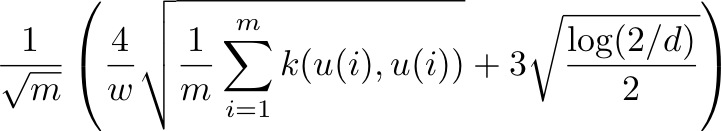.

(we take this from Theorem 7.22 of “Kernel Methods for Pattern Analysis, page 215).

If we further assume the expected value k(u,u) under the training distribution exists (i.e. we don’t have too many examples of very high kernel norm according to the distribution we are training with respect to) and is stable (not taking a lot of value from rare instances) then we can read off the meaning of this upper bound. Assuming we can always build a model from the training data of margin at least w then the generalization error (excess error we expect to see on classifying similar points) is falling at a rate proportional to the square root of the amount of training data we have. This is in one sense amazing- we have a bound on how well we are going to do on data we have not seen. And this bound is also in some sense “best possible” because we need around this much data to even confirm such an accuracy (see What is a large enough random sample? ). Also notice how the dimension of the implicit features space (which can in fact be infinite) does not enter into the bound.

However, the bound is also some sense to be expected. It depends on the following strong assumptions (usually not remembered or stated):

1. Test and training data must be generated in the same manner (i.e. come from the same distribution, this is usually stated but not obeyed).
2. We must not have collapsing margin as the number of data points goes up (so there must actually be a moat between the positive and negative examples such as portrayed in figure1 where the blurry boundary was a region we never generated training or test examples from).
3. The expected value of k(u,u) must be bounded for examples drawn from our training distribution. For example this is not true even for the identity kernel if x is just numbers drawn from a Cauchy distribution.

Also it is a posterior estimate of expected generalization error. That is, we only get a bound after we plug in some facts found from fitting a model to the training data (the margin and the expected value of k(u,u)). It is not like the famous prior bounds based on VC dimension that depend only on the structure of the kernel k(,) and not the distribution of training data or labels (beyond the concept being expressible by the kernel, which is itself a posterior fact). The thing to remember for all posterior estimates is you can get such estimates at a small expense in statistical efficiency (i.e. you waste some data) and a moderate computational blow-up by cross validation (and with essentially no math and many fewer assumptions). In fact the cross validation and hold-out estimates may be much tighter than calculated bounds (and are certainly easier to explain).

The result remains important: support vector machines are in some sense an optimal learning procedure (up to some constants they achieve as tight a generalization error as can even be confirmed for a given training set size). Another strong point of the support vector result is the result applies even for kernels with unbounded VC dimension (such as the Gaussian kernel).

## The Gaussian (or radial) kernel again

We said the Gaussian kernel was of infinite VC dimension. But we have not shown why it is true. The fact that the only encoding we could think of off the top of our head was a power-series or an limit does not guarantee there isn’t some more clever encoding that easily demonstrates that the Gaussian kernel is of bounded VC dimension (like the identity kernel and cosine kernels are).

However we can see the Gaussian kernel can not have finite VC dimension because it can place an arbitrary number of bounded patches in the plane. Thus we can build arbitrarily large sets (by placing all points far apart) that can be shattered (thus falsifying any bounded VC dimension).

One of the wonders of the support vector method is that the theory works even in this situation.

## Back to SVM

Support vector machines seem nearly magical in their power to pick “best weights.” However, as we have seen, these best weights may not always be much better than typical good weights (for instance: using all uniform weights). Also it is very important to remember the support vector machine is picking the best weighting of a sum of already picked kernels. It is not picking the best kernel shape or adjusting things like bandwidth (you must pick the best kernel ahead of time or try several kernels to find a good one). You must remember support vector machines can only directly adjust dual weights (or relative training data weighting) this can affect some changes in hypothesis shape but the support vector machine can not directly implement arbitrary changes in hypothesis shape like a nearest neighbor or a parameterized primal method can (like logistic regression with the kernel trick of adding interaction variables).

Support vector machines are to be much preferred to other combiners like Boosting. This is because support vector machines have explicit stopping criteria (conditions known to be true at the optimal solution that characterize the optimal solution) and explicit regularization (control of over fitting). Boosting relies a path argument (“do the computation in these stages”) so it is much harder to reason about and frankly the folklore that “early stop prevents over fitting in boosting” is just false (you are much better off explicitly regularizing).

It is also lore that random kernel transformations (like power series over the cosine kernel) are magic. They can make data separable but they are unlikely to meet the (unstated but critical) conditions of the support vector theorem (margin being wide, margin not filling in and expectation of k(.,.) being small).

### A comment on optimization

In this writeup we have skipped the most beautiful part of support vector machines- the form of the optimization problem used to solve them. We strongly suggest consulting John Shawe-Taylor and Nello Cristianini’s “Kernel Methods for Pattern Analysis”, Cambridge 2004 for this. The writing is dense but accurate. You can literally paste their formulas into a general solver and they work.

## Conclusion

We have shown how kernel methods and support vector machines fit in a sequence of machine learning methods:

1. nearest neighbor models
2. uniform sum models
3. support vector machines.

We also showed a number of examples of kernels and non-kernels. It is good to be able to quickly remember the constant “-1” is not a kernel and the constant “1” is a kernel (though not a very useful one).

It is our argument that support vector machines are “dual methods” (as they work in weights over the data instead of weights over coordinates in the features space) and the move from the primal algorithm Naive Bayes to Logistic regression is similar to the move from uniform kernel sums to support vector machines.

Also, if you want to directly manipulate shape (like picking bandwidth) you must in some sense use a primal method (this isn’t what support vector machines are for).

We end with: If you have some feeling of both what a method can and can not do then you have some understanding of the method.### jmount

Data Scientist and trainer at Win Vector LLC. One of the authors of Practical Data Science with R.

### 2 replies ›

1.shoonya says:

Great Article. Did you use R or Weka for the above?
I have not used R a lot for SVM, however I have used Weka quite a bit for this.

Do you have code for the above. Doing is far better learning.

2.jmount says:

@shoonya
Thanks! I used R and ggplot2 for all of the plots. For the SVM steps I just pasted the formulas from “Kernels Methods for Pattern Analysis” into http://www.win-vector.com/blog/2010/06/automatic-differentiation-with-scala/ and let a conjugate gradient optimizer do the work (not recommended for real sized problems).